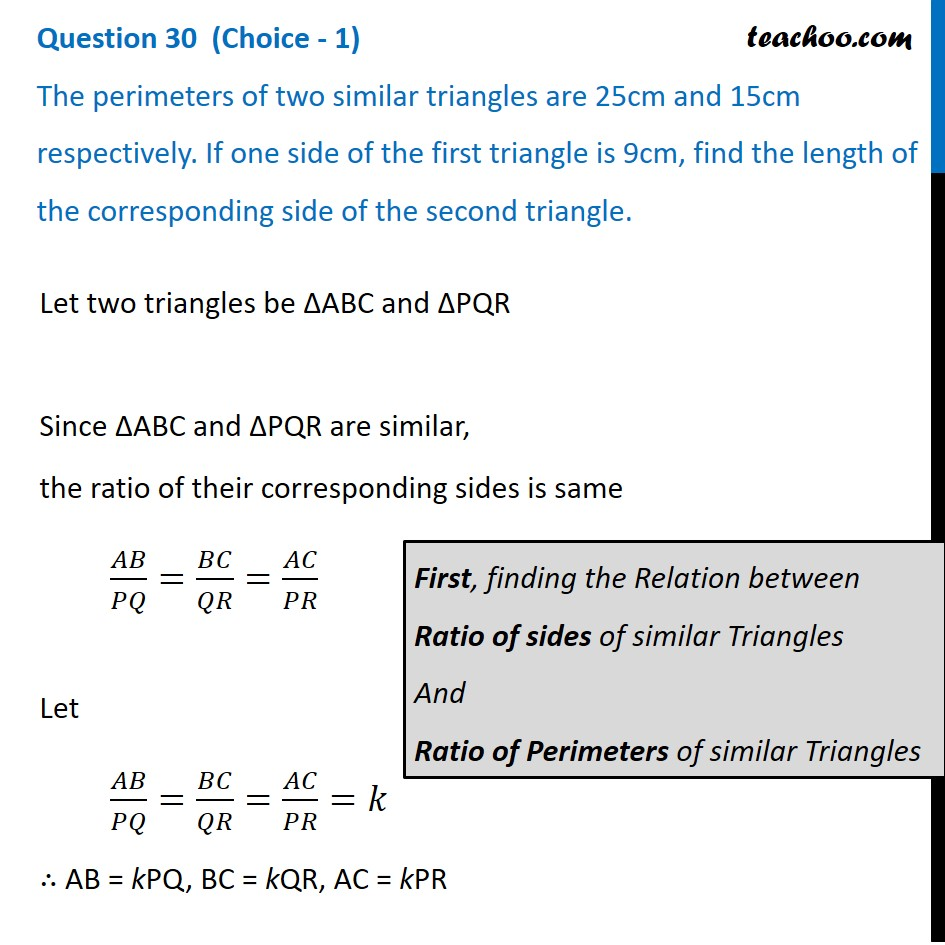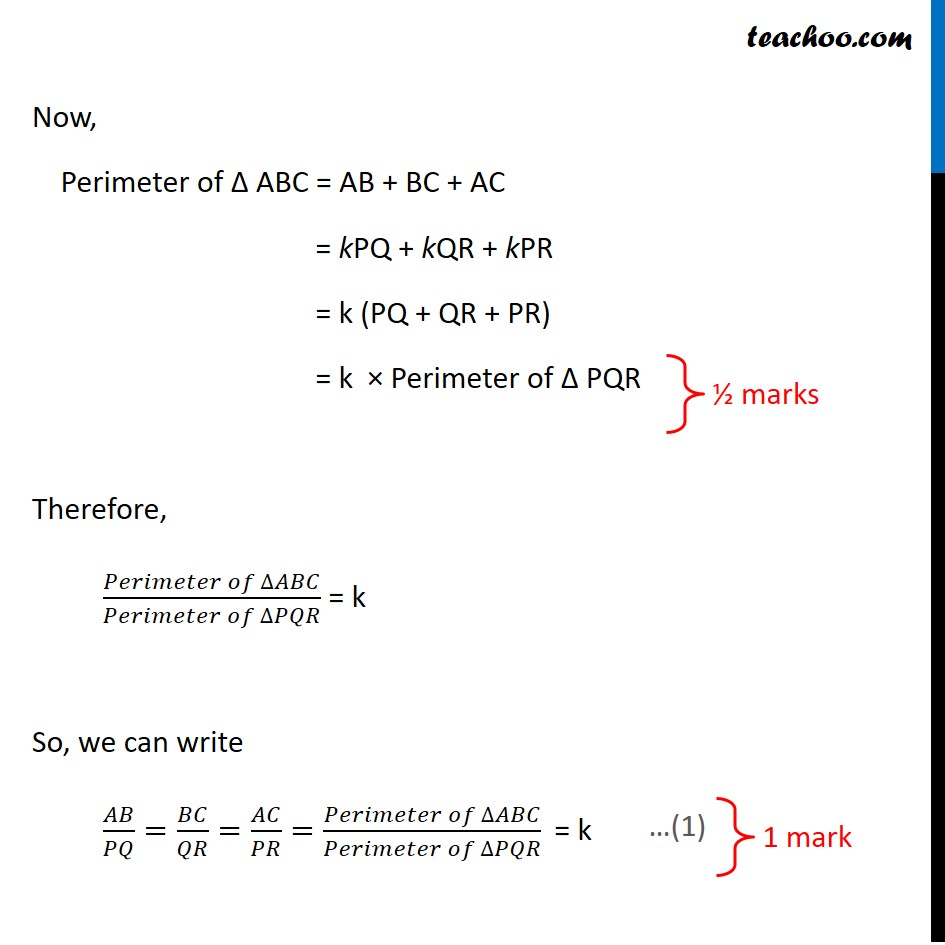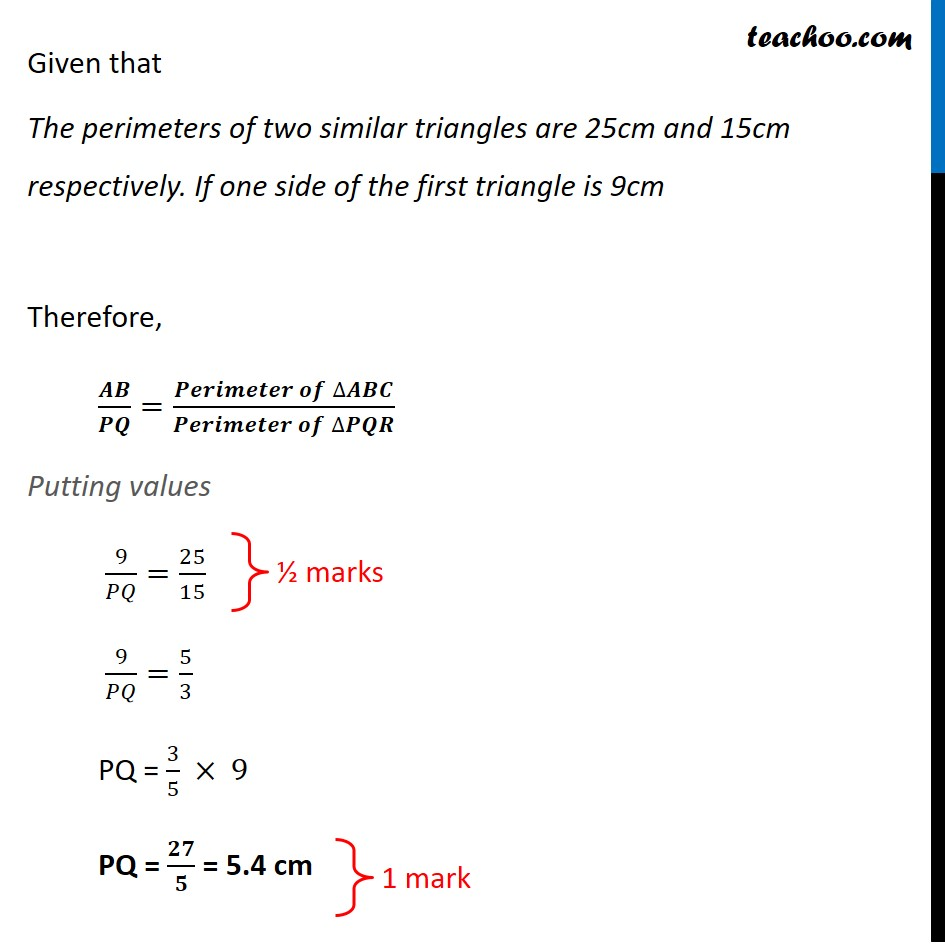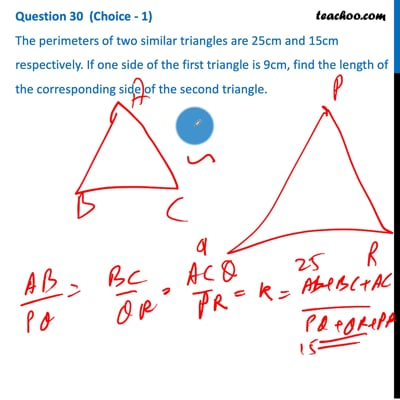CBSE Class 10 Sample Paper for 2021 Boards - Maths Standard

Class 10
Solutions of Sample Papers for Class 10 Boards

## The perimeters of two similar triangles are 25cm and 15cm respectively. If one side of the first triangle is 9cm, find the length of the corresponding side of the second triangle.This video is only available for Teachoo black users

Note : This is similar to Question 13 – Maths Standard Sample Paper 2020

Check the answer here https:// www.teachoo.com /10979/3130/Question-13/category/CBSE-Class-10-Sample-Paper-for-2020-Boards--- Maths -Standard/

Introducing your new favourite teacher - Teachoo Black, at only ₹83 per month

### Transcript

Question 30 (Choice - 1) The perimeters of two similar triangles are 25cm and 15cm respectively. If one side of the first triangle is 9cm, find the length of the corresponding side of the second triangle.Let two triangles be ΔABC and ΔPQR Since ΔABC and ΔPQR are similar, the ratio of their corresponding sides is same 𝐴𝐵/𝑃𝑄=𝐵𝐶/𝑄𝑅=𝐴𝐶/𝑃𝑅 Let 𝐴𝐵/𝑃𝑄=𝐵𝐶/𝑄𝑅=𝐴𝐶/𝑃𝑅=𝑘 ∴ AB = kPQ, BC = kQR, AC = kPR First, finding the Relation between Ratio of sides of similar Triangles And Ratio of Perimeters of similar Triangles Now, Perimeter of Δ ABC = AB + BC + AC = kPQ + kQR + kPR = k (PQ + QR + PR) = k × Perimeter of Δ PQR Therefore, (𝑃𝑒𝑟𝑖𝑚𝑒𝑡𝑒𝑟 𝑜𝑓" " ∆𝐴𝐵𝐶)/(𝑃𝑒𝑟𝑖𝑚𝑒𝑡𝑒𝑟 𝑜𝑓" " ∆𝑃𝑄𝑅) = k So, we can write 𝐴𝐵/𝑃𝑄=𝐵𝐶/𝑄𝑅=𝐴𝐶/𝑃𝑅=(𝑃𝑒𝑟𝑖𝑚𝑒𝑡𝑒𝑟 𝑜𝑓" " ∆𝐴𝐵𝐶)/(𝑃𝑒𝑟𝑖𝑚𝑒𝑡𝑒𝑟 𝑜𝑓" " ∆𝑃𝑄𝑅) " = k " Given that The perimeters of two similar triangles are 25cm and 15cm respectively. If one side of the first triangle is 9cm Therefore, 𝑨𝑩/𝑷𝑸=(𝑷𝒆𝒓𝒊𝒎𝒆𝒕𝒆𝒓 𝒐𝒇" " ∆𝑨𝑩𝑪)/(𝑷𝒆𝒓𝒊𝒎𝒆𝒕𝒆𝒓 𝒐𝒇" " ∆𝑷𝑸𝑹) " " Putting values 9/𝑃𝑄=25/15 9/𝑃𝑄=5/3 PQ = 3/5 × 9 PQ = 𝟐𝟕/𝟓 = 5.4 cm